## Friday, 8 August 2014

### CHAPTER 22 -Worked Out Examples

 Example: 23
 Prove that the area of a quadrilateral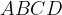$ABCD$ can be given by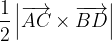$\dfrac{1}{2}\left| {\overrightarrow {AC} \times \overrightarrow {BD} } \right|$
 Solution: 23The vector area of the quadrilateral can be written as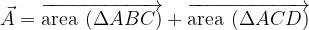$\vec A = \overrightarrow {{\rm{area}}\;\,(\Delta ABC)} + \overrightarrow {{\rm{area}}\;\,(\Delta ACD)}$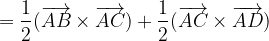$= \dfrac{1}{2}(\overrightarrow {AB} \times \overrightarrow {AC} ) + \dfrac{1}{2}(\overrightarrow {AC} \times \overrightarrow {AD} )$ To add the areas their directions must be the same$= \dfrac{1}{2}(\overrightarrow {AB} \times \overrightarrow {AC} + \overrightarrow {AC} \times \overrightarrow {AD} )$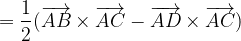$= \dfrac{1}{2}(\overrightarrow {AB} \times \overrightarrow {AC} - \overrightarrow {AD} \times \overrightarrow {AC} )$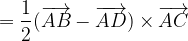$= \dfrac{1}{2}(\overrightarrow {AB} - \overrightarrow {AD} ) \times \overrightarrow {AC}$$= \dfrac{1}{2}\;\overrightarrow {DB} \times \overrightarrow {AC}$
Thus,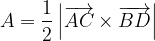$A = \dfrac{1}{2}\left| {\overrightarrow {AC} \times \overrightarrow {BD} } \right|$
 Example: 24
 Find the perpendicular distance of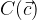$C(\vec c)$ from the segment joining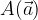$A(\vec a)$ and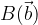$B(\vec b)$, in terms of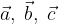$\vec a,\;\vec b,\;\vec c$.
 Solution: 24Now,
 area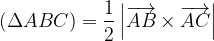$(\Delta ABC) = \dfrac{1}{2}\left| {\overrightarrow {AB} \times \overrightarrow {AC} } \right|$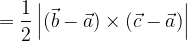$= \dfrac{1}{2}\left| {(\vec b - \vec a) \times (\vec c - \vec a)} \right|$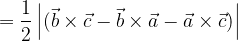$= \dfrac{1}{2}\left| {(\vec b \times \vec c - \vec b \times \vec a - \vec a \times \vec c)} \right|$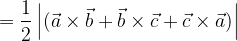$= \dfrac{1}{2}\left| {(\vec a \times \vec b + \vec b \times \vec c + \vec c \times \vec a)} \right|$
But area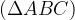$(\Delta ABC)$ also equals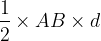$\dfrac{1}{2} \times AB \times d$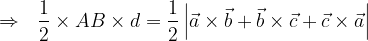$\Rightarrow \,\,\,\, \dfrac{1}{2} \times AB \times d = \dfrac{1}{2}\left| {\vec a \times \vec b + \vec b \times \vec c + \vec c \times \vec a} \right|$
Since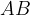$AB$ equals , we have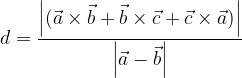$d = \dfrac{{\left| {(\vec a \times \vec b + \vec b \times \vec c + \vec c \times \vec a)} \right|}}{{\left| {\vec a - \vec b} \right|}}$